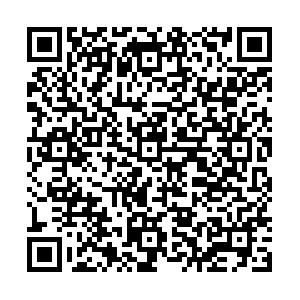EI、Scopus 收录

## 留言板引用本文: 吴华, 邹绍华, 徐成辉, 尉亚军, 邓子辰. 广义热弹模型的热力学基础与瞬态响应. 力学学报, 2022, 54(11): 1-12Wu Hua, Zou Shaohua, Xu Chenghui, Yu Yajun, Deng Zichen. Thermodynamic basis of generalized thermoelasticity and transient response. Chinese Journal of Theoretical and Applied Mechanics, 2022, 54(11): 1-12 doi: 10.6052/0459-1879-22-225
 Citation: Wu Hua, Zou Shaohua, Xu Chenghui, Yu Yajun, Deng Zichen. Thermodynamic basis of generalized thermoelasticity and transient response. Chinese Journal of Theoretical and Applied Mechanics, 2022, 54(11): 1-12## THERMODYNAMIC BASIS OF GENERALIZED THERMOELASTICITY AND TRANSIENT RESPONSE

• 摘要: 微纳科技的快速发展与超短脉冲激光技术的广泛运用, 对描述微纳尺度超快热冲击的广义热传导及其热弹耦合理论提出迫切需求. 基于拓展热力学原理, 本文建立了考虑热传导双相滞后效应和高阶热流率的广义热弹耦合理论. 类比于力学领域黏弹性本构关系的串联、并联模型, 并受Green-Naghdi (GN)广义热传导模型启发, 本文提出了热学“弹性”单元和“黏性”单元模型, 并采用串联、并联方法实现了Cattaneo-Vernotte (CV)、GN、双相滞后(DPL)和Moore-Gibson-Thompson (MGT) 热传导模型的重构. 理论推导进一步表明, 本文新建模型对应于热学Burgers模型, 并得到了新模型中各相位滞后中松弛时间之间的比例关系. 运用拉普拉斯变换方法, 研究了一维结构受边界热冲击和移动热源作用下的瞬态响应, 计算结果表明: 新模型克服了热波速度无限大的悖论; 仅有边界热冲击载荷时, 新模型得到的响应结果均较大, 响应范围最小; 相比于无热源作用情形, 受移动热源作用时, 新模型会产生更大的峰值响应. 新模型与经典弹性理论耦合构建了广义热弹性理论, 运用该理论, 可以清晰观察到在热波和弹性波波前的应力突变. 理论方面, 本文推动了拓展热力学与连续介质力学的结合, 对于远离平衡态极端力学基础理论问题的研究具有启发意义; 应用方面, 本文研究结果可为激光等移动热源作用下材料的瞬态响应分析提供理论基础和数值方法.

• 图  1  热学黏弹性单元组合模型

Figure  1.  Combination model of thermovisco and thermoelastic elements

图  2  无热源下各模型位移、应力、温度响应

Figure  2.  Displacement, stress and temperature responses of each model without heat source

图  3  移动热源下各模型的位移、应力、温度响应

Figure  3.  Displacement, stress and temperature responses of each model under moving heat source

图  4  移动热源下DPL模型和新模型位移、应力、温度的时间演化特征

Figure  4.  Evolution laws of displacement, stress and temperature of DPL model and new model under moving heat source

表  1  广义热传导模型的变换和统一化

Table  1.   Transformation and unification of generalized heat conduction models

 Heat Conduction Equation Transformed Equation $\bar M$ $\bar N$ CV $q + \tau \dot q = - k\nabla T$ $q + \tau sq = - k\nabla T$ $1 + \tau s$ 1 GN $\tau \dot q = - k\nabla T - k\tau \nabla \dot T$ $\tau sq = - k\nabla T - k\tau s\nabla T$ $\tau s$ $1 + \tau s$ DPL $q + 2\tau \dot q = - k\nabla T - k\tau \nabla \dot T$ $q + 2\tau sq = - k\nabla T - k\tau s\nabla T$ $1 + 2\tau s$ $1 + \tau s$ MGT $2\tau \dot q + {\tau ^2}\ddot q = - k\nabla T - k\tau \nabla \dot T$ $2\tau sq + {\tau ^2}{s^2}q = - k\nabla T - k\tau s\nabla T$ $2\tau s + {\tau ^2}{s^2}$ $1 + \tau s$ New Model $q + 3\tau \dot q + {\tau ^2}\ddot q = - k\nabla T - k\tau \nabla \dot T$ $q + 3\tau sq + {\tau ^2}{s^2}q = - k\nabla T - k\tau s\nabla T$ $1 + 3\tau s + {\tau ^2}{s^2}$ $1 + \tau s$

表  2  材料常数表(铜)

Table  2.   Material constants (Copper)

 $\lambda/ {{\text{GPa}}}$ $\mu /{{\text{GPa}}}$ ${\alpha _\theta }\left( {{\rm{m\cdot K^{-1}}}} \right)$ $\rho/\left( {{\text{kg}}\cdot{{\text{m}}^{-3}}} \right)$ ${c_E}/\left( {{\text{J}}\cdot {{\rm{kg^{-1}K^{-1}}}} } \right)$ ${T_0}/{\text{K}}$ 77.6 38.6 1.78 e-5 8945 381 293
•点击查看大图
##### 计量
• 文章访问数:  43
• HTML全文浏览量:  8
• PDF下载量:  13
• 被引次数: 0
##### 出版历程
• 收稿日期:  2022-05-27
• 录用日期:  2022-09-16
• 网络出版日期:  2022-09-17

### 目录/下载:  全尺寸图片 幻灯片
• 分享
• 用微信扫码二维码

分享至好友和朋友圈